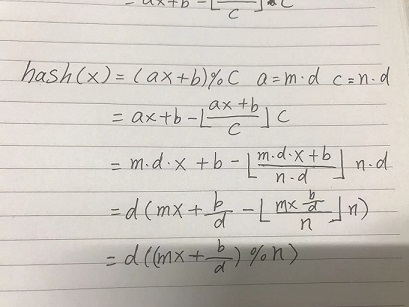# 概述

• 哈希表是一种可以满足快速查找数据结构，时间复杂度接近O(1)。
• 哈希函数是无限集到有限集的映射。
• 处理数据量大，查找效率要求高时推荐使用hash容器。
• 问题：
• 什么情况下考虑使用哈希容器？
• 常用的哈希思路有哪些？
• 评判哈希算法标准有哪些？
• 哈希冲突是如何产生的？如何解决？
• 如何构造一个hash算法？应注意哪些问题？

• 效率高。
• 映射分布均匀。

# 基础hash思路

## 平方取中法：

key取平方，截取中间的几位作为新的key。数学计算的性质乘积中间几位和乘数每一位都有关，充分混合key每一位对生成的哈希值的影响，使映射分布更均匀。

## 取余法：

Hash(key) = key % m

## 相乘取整法:

Hash(key) = floor(frac(key * A), m), 0<A<1

• floor 取整，frac 取小数
• 此法避免像除余法中结果对m过于依赖。

## 随机数法

Hash(key) = rand(key)

• 据我所知C#的object采用此方法，使用元数据中的几位存hash值。

## 折叠法：

• 如：Hash(1234,m = 2) = 46。
• 关键字较长时可以考虑使用此方法。

# 哈希冲突

## 解决办法

### 开放地址法

Hi=（H（key）+di)Hi=（H（key）+di) % m   i=1,2,,3….,ni=1,2,,3….,n

• H(key) 为哈希函数
• m 为表长
• di 为增量序列

• 线性探测：di = 1,2,3,……
• 二次探测: di = ±1^2， ±2^2，…….
• 随机探测: di = random(di，seed)
• random 为 无状态的伪随机发生函数（所谓无状态，即无论多少次调用，random(a) = b不变）
• seed 一个确定不变的随机数种子

### 链式地址法

pos1
pos2 -> val -> val
pos3 -> val
pos4

### 再哈希法

Hi=RHi（key）i=1，2，…，k

## 哈希冲突解决方法优缺点分析

### 开放散列：链式地址法（桶链法）

• 优点:
• 添加删除方便，避免动态调整开销
• 桶链表内存动态分配，减少内存浪费
• 当哈希表size很大时，指针的性能消耗可以忽略
• 缺点：
• 动态分配内存，内存不紧凑，随机访问性差，序列化性能差。
• 对于预先知道所有元素，可以实现没有冲突的完美hash函数，此时效率会远低于封闭散列。

### 封闭散列：开放地址法，再哈希法 …

• 优点：
• 内存紧凑，随机访问性能好，序列化性能好。
• 预先知道所有元素e，可以实现完美hash函数，此时效率远高于开放散列。
• 缺点：
• 所有条目数量不能超过数组的长度，扩容/收紧频繁，性能消耗大。
• 碰撞探测消耗性能。
• 当数组长度很大时，有内存浪费。

# 哈希算法进阶实例分析

``````-- lua 5.4

unsigned int luaS_hash (const char *str, size_t l, unsigned int seed,
size_t step) {
unsigned int h = seed ^ cast_uint(l);
for (; l >= step; l -= step)
h ^= ((h<<5) + (h>>2) + cast_byte(str[l - 1]));
return h;
}

#define lmod(s,size) \
(check_exp((size&(size-1))==0, (cast_int((s) & ((size)-1)))))
``````

(h << 5) + (h >> 2)
= (((h << 5) << 2) + ((h >> 2) << 2) >> 2)
= ((h << 7) + h) >> 2
= (129 * h) >> 2

• 和伪随机数生成算法一样，要让生成的数尽量随机–二进制数的每一个位取0或1的概率都是50%。

• 移位，异或运算充分混合每一位的影响，而加法运算引起多个位的反转，使hash值的每一个位更加不可预测，以接近不可逆的单向函数。

• (h << 5) + (h >> 2) = (129 * h) >> 2。 乘法可以被拆分为加法和移位的组合（即（h << 7）+h ），以混合哈希值。不过(h << 7 – h) = 127h 会更好些，127是梅森素数（2^n -1）。与线性同余算法（LCG）生成伪随机数一样，梅森素数127，只需一次移位运算和一次加法运算，且不会被分解，随机数分布更加均匀。• 非素数会被分解成更小的素数的乘积，参与运算时容易被分解,上例中a和c可以提取公因数d，周期 = n = c/d。
• a%b = a&(b-1) 当 b = 2^n 时等式成立，lua哈希表的长度保证符合等式成立的条件，lmod使用位运算代替取余运算，效率更高。

• 算法实际应用详情请参考我的文章